# Harmonic

Calculate harmonic over period 1/f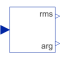# Information

This information is part of the Modelica Standard Library maintained by the Modelica Association.

This block calculates the root mean square and the phase angle of a single harmonic k of the input signal u over the given period 1/f, using the mean block.

Note: The output is updated after each period defined by 1/f.

Note:
The harmonic is defined by `√2 rms cos(k 2 π f t - arg)` if useConjugateComplex=false (default)
The harmonic is defined by `√2 rms cos(k 2 π f t + arg)` if useConjugateComplex=true

# Parameters (5)

f Value: Type: Frequency (Hz) Description: Base frequency Value: Type: Integer Description: Order of harmonic Value: false Type: Boolean Description: Gives conjugate complex result if true Value: 0 Type: Real Description: Start value of cos integrator state Value: 0 Type: Real Description: Start value of sin integrator state

# Connectors (3)

u y_rms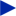Type: RealInput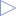Type: RealOutput Description: Root mean square of polar representationType: RealOutput Description: Angle of polar representation

# Components (8)

sin1 sin2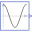Type: Cosine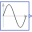Type: Sine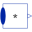Type: MultiProductType: MultiProduct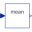Type: MeanType: Mean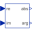Type: RectangularToPolar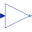Type: Gain

# Used in Examples (5)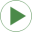MultiPhaseTwoLevel_R Modelica.Electrical.PowerConverters.Examples.DCAC.MultiPhaseTwoLevel Multi phase DC to AC converter with R loadMultiPhaseTwoLevel_RL Modelica.Electrical.PowerConverters.Examples.DCAC.MultiPhaseTwoLevel Multi phase DC to AC converter with R-L loadThreePhaseTwoLevel_PWM Modelica.Electrical.PowerConverters.Examples.DCAC.MultiPhaseTwoLevel Test of pulse width modulation methodsRectifier Modelica.Electrical.QuasiStationary.SinglePhase.Examples Rectifier exampleNonLinearInductor Modelica.Magnetic.QuasiStatic.FluxTubes.Examples Non linear inductor with ferromagnetic core

# Used in Components (3)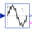TotalHarmonicDistortion Modelica.Blocks.Math Output the total harmonic distortion (THD)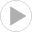SinglePhaseTwoLevel Modelica.Electrical.PowerConverters.Examples.DCAC.ExampleTemplates Single phase two level inverter including control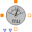FundamentalWavePermabilitySensor Modelica.Magnetic.QuasiStatic.FluxTubes.Sensors.Transient Sensor of fundamental wave permeability